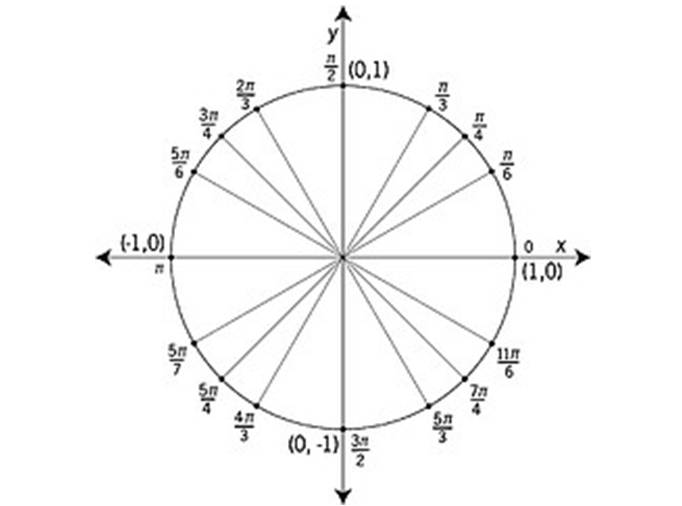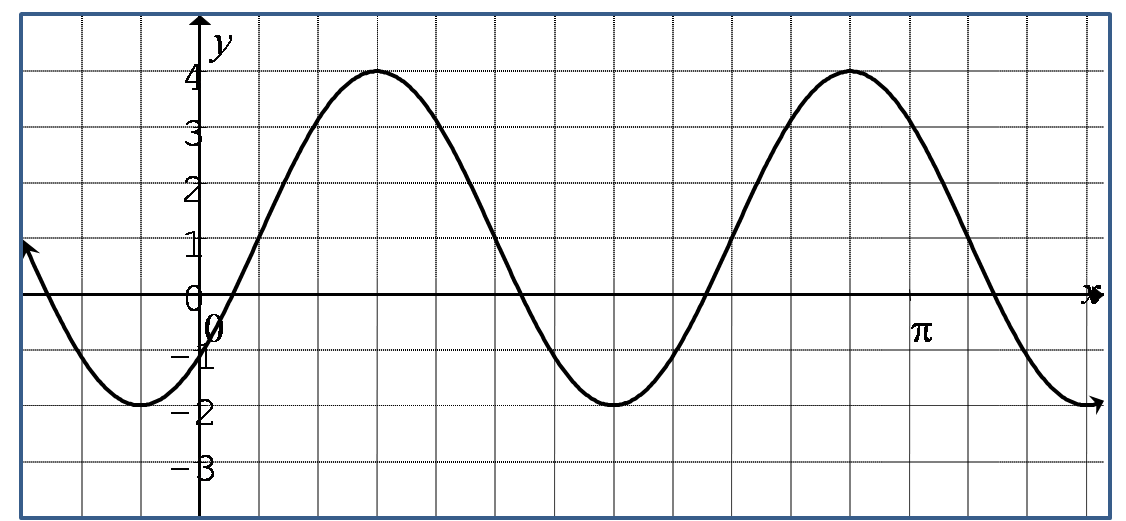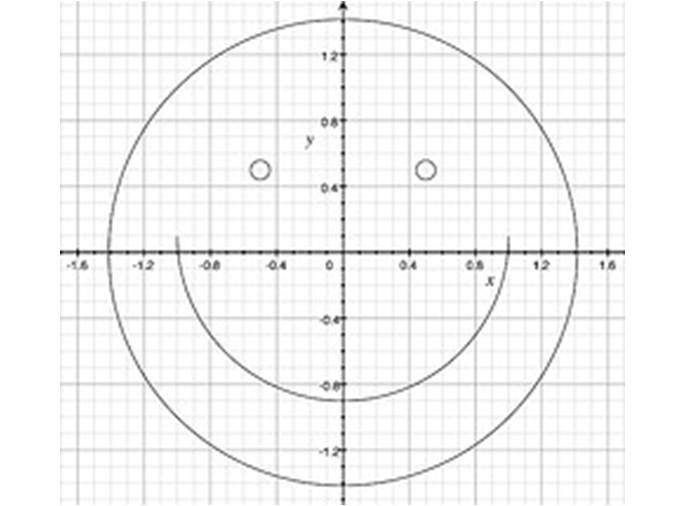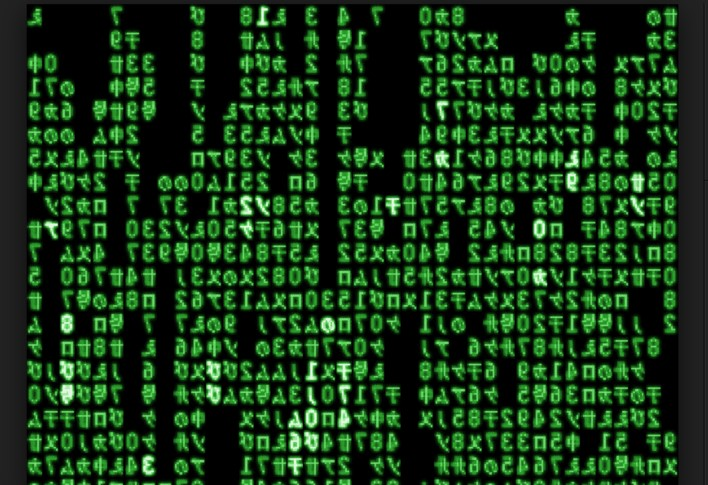﻿ Math 12 Honours
 FAIL (the browser should render some flash content, not this).Math 12 Honours Ch 1

Functions and Transformation

In this chapter, students will learn:

• Translate a function horizontally and vertically
• Reflection a function over both axis
• Expand and compress a function horizontally and vertically
• Graph the inverse of a function

Click for Homework

Notes

•  1.1
•  1.2
•  1.3
•  1.4
•  1.5
•  1.6
•  RevAt BCMath.ca we offer hundreds of free online math lessons for students and teachers. Help support our site by clicking on the button above to donate through Paypal.Math 12 Honours Ch 2

In this chapter, students will learn:

• Apply transformations to Trigonometric functions
• Solve advanced trigonometric equations using identities and transformations
• Explore basic applications of trigonometric equations

Click for Homework

Notes

•  2.1
•  2.2
•  2.3
•  2.4
•  2.5
•  2.6
•  2.7
•  2.8
•  2.9
•  2.10
•  2.11
•  2.12Trigonometric Functions

In this chapter, students will learn:

• Apply transformations to Trigonometric functions
• Solve advanced trigonometric equations using identities and transformations
• Explore basic applications of trigonometric equations

Click for Homework

Notes

•  3.1
•  3.2
•  3.3
•  3.4
•  3.5
•  3.6
•  3.7
•  3.8
•  3.9Math 12 Honours Ch 4

Logarithms and Exponentials

In this chapter, students will learn:

• to solve basic equations involving exponents and logarithms
• to graph logarithmic and exponential functions with transformations
• basic applications of logarithm and exponents
• Solve advanced equations with Logarithm identities

Notes

•  4.1
•  4.2
•  4.3
•  4.4
•  4.5
•  4.6
•  4.7Math 12 Honours Ch 5

Relations and Conics

In this chapter, students will learn:

• to prove basic geometry concents with coordinate geometry
• to graph Circles, Ellipses, and Hyperbolas with transformations
• to calculate the shortest distance from any point to a line

Notes

•  5.1
•  5.2
•  5.3
•  5.4
•  5.5
•  5.6Math 12 Honours Ch 6

•  Section 6.1: What are Matrices
•  Section 6.2: Applications of Matrices
•  Section 6.2: Vectors
•  Section 6.2: Applications of Matrices
•  Chapter 6 Review

Linear Algebra and Matrices

In this chapter, students will learn:

• Basic properties of Matrices
• Applications of Matrices in Linear Algebra

•  6.1
•  6.2
•  6.3
•  6.4
•  6.5
•  6.6Math 12 Honours Ch 7

•  Section 7.1: What are Limits
•  Section 7.1: What are Limits

Limits

In this chapter, students will learn:

• how to calculate the limit of a function
• Applications of Limits

•  7.1
•  7.2
•  7.3
•  7.4
•  7.5
•  7.6
•  7.7Math 12 Honours Ch 8

•  Section 8.1: Functions & Transformations
•  Section 8.2: Logarithms & Exponentials
•  Section 8.3: Trigonometric Functions
•  Section 8.4: Graphing Trigonometric Functions

Statistics

•  Section 8.5: Trigonometric Identities
•  Section 8.6: Counting Principles
•  Section 8.7: Probability
•  Section 8.8: Statistics
•  Section 8.9: Circles, Ellipses, and ConicsMath 12 Honours Ch 9

•  Section 9.1: Functions & Transformations
•  Section 9.2: Logarithms & Exponentials
•  Section 9.3: Trigonometric Functions
•  Section 9.4: Graphing Trigonometric Functions

Circles, Ellipses, and Conics

•  Section 9.5: Trigonometric Identities
•  Section 9.6: Counting Principles
•  Section 9.7: Probability
•  Section 9.8: Statistics
•  Section 9.9: Circles, Ellipses, and Conics